# Curvature is tensorial

This article gives the statement, and possibly proof, that a map constructed in a certain way is tensorial
View other such statements

## Statement

Let$\nabla$ be a connection on a vector bundle$E$ over a differential manifold$M$. The Riemann curvature tensor of$\nabla$ is given as a map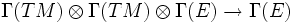$\Gamma(TM) \otimes \Gamma(TM) \otimes \Gamma(E) \to \Gamma(E)$ defined by: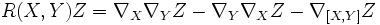$R(X,Y)Z = \nabla_X\nabla_Y Z - \nabla_Y \nabla_X Z - \nabla_{[X,Y]} Z$

We claim that$R$ is a tensorial map in each of the variables$X,Y,Z$.

## Facts used

Fact no. Name Statement with symbols
1 Any connection is$C^\infty$-linear in its subscript argument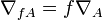$\nabla_{fA} = f\nabla_A$ for any$C^\infty$-function$f$ and vector field$A$.
2 The Leibniz-like axiom that is part of the definition of a connection For a function$f$ and vector fields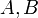$A,B$, and a connection$\nabla$, we have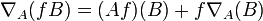$\nabla_A(fB) = (Af)(B) + f\nabla_A(B)$
3 Corollary of Leibniz rule for Lie bracket (in turn follows from Leibniz rule for derivations For a function$f$ and vector fields$X,Y$: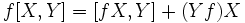$\! f[X,Y] = [fX,Y] + (Yf)X$$\! f[X,Y] = [X,fY] - (Xf)Y$

## Proof

To prove tensoriality in a variable, it suffices to show$C^\infty$-linearity in that variable. This is because linearity in$C^\infty$-functions guarantees linearity in a function that is 1 at exactly one point, and zero at others.

The proofs for$X$ and$Y$ are analogous, and rely on manipulation of the Lie bracket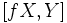$[fX,Y]$ and the property of a connection being$C^\infty$ in the subscript vector. These proofs do not involve any explicit use of$Z$. The proof for$Z$ relies simply on repeated application of the product rule, and the fact that$XY - YX = [X,Y]$.

### Tensoriality in the first variable

Given: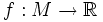$f:M \to \R$ is a$C^\infty$-function.

To prove: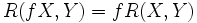$\! R(fX,Y) = f R(X,Y)$, or more explicitly,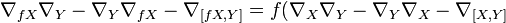$\! \nabla_{fX}\nabla_Y - \nabla_Y \nabla_{fX} - \nabla_{[fX,Y]} = f(\nabla_X\nabla_Y - \nabla_Y\nabla_X - \nabla_{[X,Y]}$

We start out with the left side: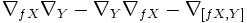$\nabla_{fX}\nabla_Y - \nabla_Y \nabla_{fX} - \nabla_{[fX,Y]}$

Each step below is obtained from the previous one via some manipulation explained along side.

Step no. Current status of left side Facts/properties used Specific rewrites
1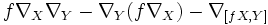$f\nabla_X\nabla_Y - \nabla_Y (f \nabla_X) - \nabla_{[fX,Y]}$ Fact (1):$\nabla$ is$C^\infty$-linear in its subscript argument.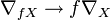$\nabla_{fX} \to f\nabla_X$
2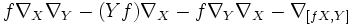$f\nabla_X\nabla_Y - (Yf)\nabla_X - f \nabla_Y\nabla_X - \nabla_{[fX,Y]}$ Fact (2)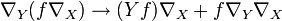$\nabla_Y(f \nabla_X) \to (Yf)\nabla_X + f\nabla_Y\nabla_X$. To understand this more clearly imagine an input$Z$ to the whole expression, so that the rewrite becomes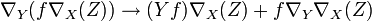$\nabla_Y(f \nabla_X(Z)) \to (Yf)\nabla_X(Z) + f\nabla_Y\nabla_X(Z)$. In the notation of fact (3),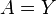$A = Y$,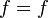$f = f$, and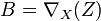$B = \nabla_X(Z)$.
3$f(\nabla_X\nabla_Y - \nabla_Y\nabla_X) - \nabla_{(Yf)X} - \nabla_{[fX,Y]}$ Fact (1)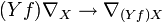$(Yf)\nabla_X \to \nabla_{(Yf)X}$
4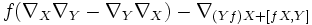$f(\nabla_X\nabla_Y - \nabla_Y\nabla_X) - \nabla_{(Yf)X + [fX,Y]}$$\nabla$ is additive in its subscript argument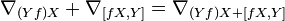$\nabla_{(Yf)X} + \nabla_{[fX,Y]} = \nabla_{(Yf)X + [fX,Y]}$
5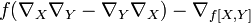$f(\nabla_X\nabla_Y - \nabla_Y\nabla_X) - \nabla_{f[X,Y]}$ Fact (3)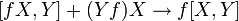$[fX,Y] + (Yf)X \to f[X,Y]$
6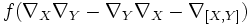$f(\nabla_X\nabla_Y - \nabla_Y\nabla_X - \nabla_{[X,Y]})$ Fact (1)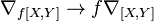$\nabla_{f[X,Y]} \to f\nabla_{[X,Y]}$

### Tensoriality in the second variable

Given:$f:M \to \R$ is a$C^\infty$-function.

To prove: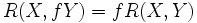$\! R(X,fY) = f R(X,Y)$, or more explicitly,$\nabla_X\nabla_{fY} - \nabla_{fY}\nabla_X - \nabla_{[X,fY]} = f (\nabla_X\nabla_Y - \nabla_Y\nabla_X - \nabla_{[X,Y]}$.

We start out with the left side: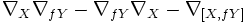$\nabla_X\nabla_{fY} - \nabla_{fY}\nabla_X - \nabla_{[X,fY]}$

Each step below is obtained from the previous one via some manipulation explained along side.

Step no. Current status of left side Facts/properties used Specific rewrites
1$\nabla_X(f\nabla_Y) - f\nabla_Y\nabla_X - \nabla_{[X,fY]}$ Fact (1)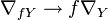$\nabla_{fY} \to f\nabla_Y$.
2$(Xf)\nabla_Y + f(\nabla_X\nabla_Y) - f\nabla_Y\nabla_X - \nabla_{[X,fY]}$ Fact (2)$\nabla_X(f\nabla_Y) \to (Xf)\nabla_Y + f(\nabla_X\nabla_Y)$. To make this more concrete, imagine an input$Z$. Then, the rewrite becomes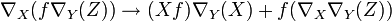$\nabla_X(f\nabla_Y(Z)) \to (Xf)\nabla_Y(X) + f(\nabla_X\nabla_Y(Z))$. This comes setting$A = X$,$f = f$,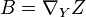$B = \nabla_YZ$ in Fact (3).
3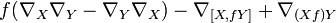$f(\nabla_X\nabla_Y - \nabla_Y\nabla_X) - \nabla_{[X,fY]} + \nabla_{(Xf)Y}$ Fact (1)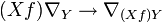$(Xf)\nabla_Y \to \nabla_{(Xf)Y}$
4$f(\nabla_X\nabla_Y - \nabla_Y\nabla_X) - \nabla_{[X,fY] - (Xf)Y}$$\nabla$ is additive in its subscript argument.$\nabla_{[X,fY]} - \nabla_{(Xf)Y} \to \nabla_{[X,fY] - (Xf)Y}$.
5$f(\nabla_X\nabla_Y - \nabla_Y\nabla_X) - \nabla_{f[X,Y]}$ Fact (3)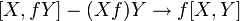$[X,fY] - (Xf)Y \to f[X,Y]$
6$f(\nabla_X\nabla_Y - \nabla_Y\nabla_X - \nabla_{[X,Y]})$ Fact (1)$\nabla_{f[X,Y]} \to f\nabla_{[X,Y]}$

### Tensoriality in the third variable

Given: A$C^\infty$-function$f:M \to \R$.

To prove:$\! R(X,Y) (fZ) = f R(X,Y) Z$. More explicitly,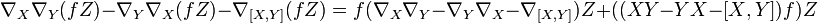$\! \nabla_X\nabla_Y(fZ) - \nabla_Y\nabla_X(fZ) - \nabla_{[X,Y]}(fZ) = f (\nabla_X\nabla_Y - \nabla_Y\nabla_X - \nabla_{[X,Y]})Z + ((XY - YX - [X,Y])f)Z$.

We start out with the left side: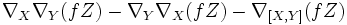$\nabla_X\nabla_Y(fZ) - \nabla_Y\nabla_X(fZ) - \nabla_{[X,Y]}(fZ)$

Each step below is obtained from the previous one via some manipulation explained along side.

Step no. Current status of left side Facts/properties used Specific rewrites
1$\! \nabla_X( (Yf)(Z) + f \nabla_YZ) - \nabla_Y ((Xf)Z + f \nabla_XZ) - f \nabla_{[X,Y]}Z - ([X,Y]f) Z$ Fact (2)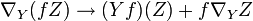$\nabla_Y(fZ) \to (Yf)(Z) + f\nabla_YZ$ and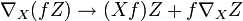$\nabla_X(fZ) \to (Xf)Z + f\nabla_XZ$
2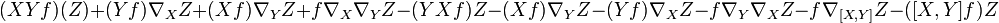$\! (XYf)(Z) + (Yf) \nabla_XZ + (Xf) \nabla_YZ + f \nabla_X\nabla_YZ - (YXf)Z - (Xf) \nabla_YZ - (Yf) \nabla_XZ -f \nabla_Y\nabla_XZ - f \nabla_{[X,Y]}Z - ([X,Y]f) Z$ Fact (2)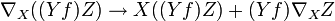$\nabla_X((Yf)Z) \to X((Yf)Z) + (Yf)\nabla_XZ$, etc.
3$f (\nabla_X\nabla_Y - \nabla_Y\nabla_X - \nabla_{[X,Y]})Z + ((XY - YX - [X,Y])f)Z$ -- cancellations
4$f (\nabla_X\nabla_Y - \nabla_Y\nabla_X - \nabla_{[X,Y]})Z + ((XY - YX - [X,Y])f)Z$ use$[X,Y] = XY - YX$, definition cancellation